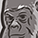cancel
Showing results for
Did you mean:New Contributor

## Molarity of KOH solution

Hello, I'm adding 18,460 grams (40.69 lbs) of 90% anhydrous dry flake KOH to 561 liters (148.4 gallons) of water.  I arrive at a molarity of 0.53 but our colleague gets 0.78.

Many thanksNew Contributor II

## Re: Molarity of KOH solution

To calculate the molarity of a solution, you need to know the moles of KOH and the volume of the water. To resolve the discrepancy between your calculation (0.53 M) and your colleague's calculation (0.78 M), let's calculate it step by step:

Calculate the moles of KOH:

You have 18,460 grams of KOH.
KOH has a molar mass of approximately 56.11 g/mol (39.10 g/mol for K and 16.00 g/mol for O).
Moles of KOH = 18,460 g / 56.11 g/mol ≈ 328.57 moles

You're dissolving this in 561 liters (561,000 mL) of water.
Molarity (M) = Moles of solute / Liters of solution
Molarity = 328.57 moles / 561,000 mL = 0.585 M (rounded to three decimal places)

So, the molarity of your KOH solution is approximately 0.585 M, not 0.53 M or 0.78 M.  🙂